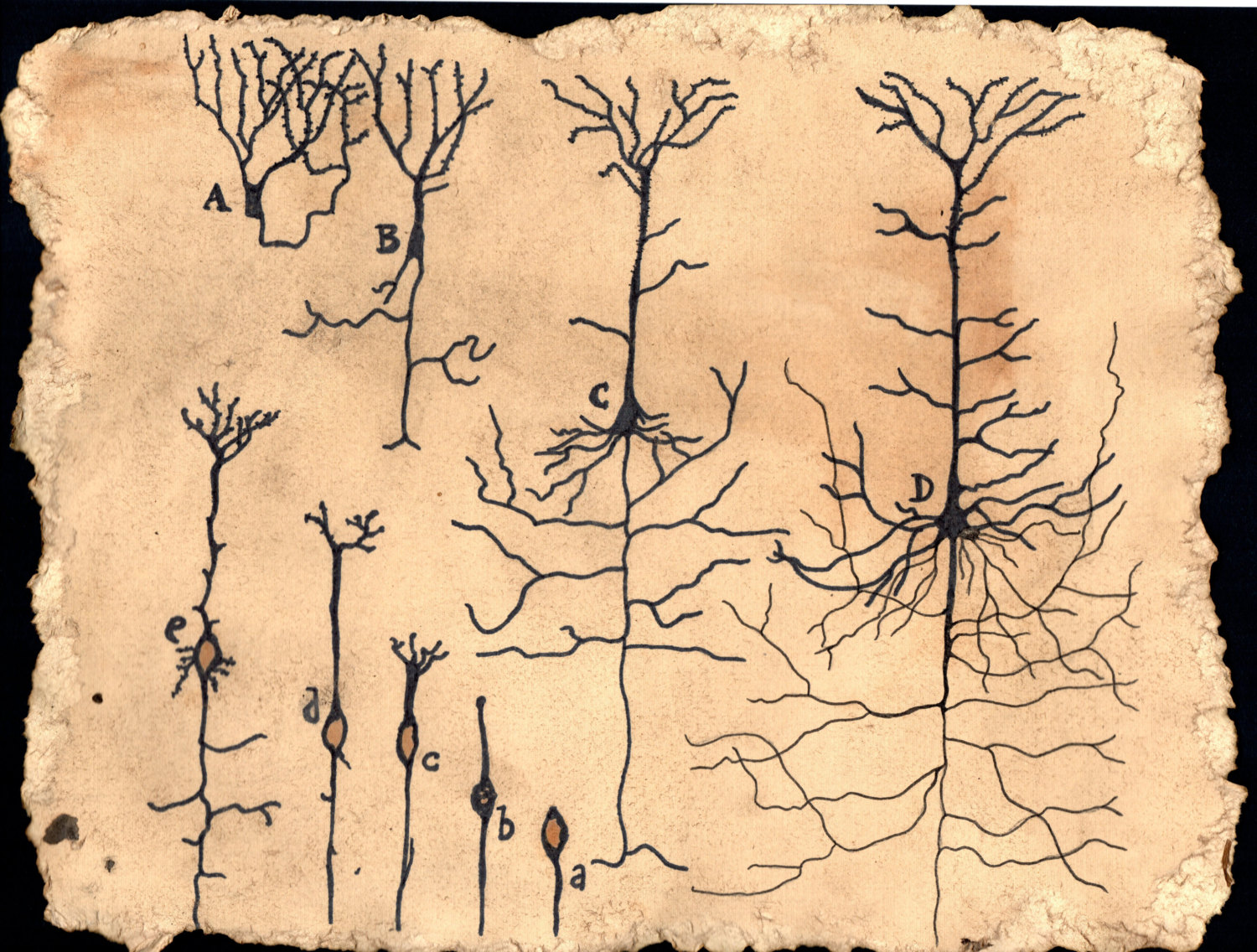Voltage in a neuron

Imagine two rooms without windows or doors. Now imagine those two rooms are connected by a single hole the size of a straw. If the first room is filled with perfume, the perfume molecules will be bouncing around the room randomly. Some of those perfume molecules will happen to travel through the hole connecting the rooms. As this happens, the number of perfume molecules in the second room will start to go up, and the number of perfume molecules in the first room will go down. Concentration is the amount of something in a volume (the room, in this case). As the concentration of perfume goes up in the second room, some of its bouncing perfume molecules travel back through the hole to the first room, but still not as fast as the perfume molecules are traveling from the first room to the second. The difference in concentration between the two rooms is the concentration gradient, and that gradient goes down as more and more perfume molecules from the first room enter the second. Eventually, the concentration of perfume molecules is the same in both rooms, and the rate at which molecules are leaving both rooms are equal. So we say the rooms have reached equilibrium.

Inside the Neuron

The situation in a neuron is the same. The membrane of every neuron is studded with proteins that control the flow of ions from one side to the other. Some of these proteins act as pores for ions to travel through. If the inside of a neuron has a higher concentration of an ion like K+ than the outside (it does, in fact), then K+ ions will bounce around in the neuron and pass through the ion channels at a greater rate than incoming K+ ions, just as in the perfume example.

Introducing Voltage

Without voltage, the K+ ions in the inside would eventually diffuse through the ion channels to the outside of the cell, until the concentration of K+ ions was the same inside and outside the cell. Voltage prevents this from happening. I assume you have some understanding of voltage, but I'll refresh your memory. Charge, like gravity, causes things to be attracted. Particles that have opposite charges are attracted to each other. Also, particles that have similar charges are repulsed from each other. We say that charge causes a force on particles. Voltage is defined as the energy required to push or pull a particle from one point to another divided by the charge of that particle. That 'divided by' is necessary, because if you have a particle of twice the positive charge, and you are pushing it towards another particle of positive charge, it would take twice as much energy. Let's clarify with an example: suppose you have an enormous metal sphere with a strongly negative charge in one hand, and a tiny crunched up ball of aluminum foil from a gum wrapper that somehow has 2 coulombs (the unit of charge) of negative charge in the other. If you push the ball of foil towards the metal sphere and you exert 4 joules of energy, then that foil has gained 4 joules, and its new location has 2 volts ($\frac{4J}{2C}$) more voltage (Energy per coulomb of charge) than its starting location.

where $E_x$ is the equilibrium potential, $R$ is the gas constant, $T$ is the temperature in kelvin, $z$ is the valence (charge) of the ion, $F$ is Faradays constant, $\left [ X \right ]_o$ is the concentration of the ion outside the cell, and $\left [ X \right ]_i$ is the concentration of the ion inside the cell. The Nernst Equation gives the equilibrium potential only when the neuron's membrane is permeable to one ion. If the neuron has ion channels for more than one ion, the equilibrium potential depends on a sum of the concentrations of the ions, weighted according to how permeable the membrane is to each ion. The final formula for determining the resting potential of a neuron is given by the Goldman Equation. Here is the equation, using the three most important ions to the resting potential: